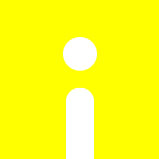opencv_ex30-彩色或灰階轉HSV格式cvtColor、灰階圖像可調式邊緣檢測Canny、從邊緣圖找輪廓的點findContours、輪廓點進行多邊形擬合approxPolyDP、求包

# opencv_ex30-彩色或灰階轉HSV格式cvtColor、灰階圖像可調式邊緣檢測Canny、從邊緣圖找輪廓的點findContours、輪廓點進行多邊形擬合approxPolyDP、求包

opencv_ex30-彩色或灰階轉HSV格式cvtColor、灰階圖像可調式邊緣檢測Canny、從邊緣圖找輪廓的點findContours、輪廓點進行多邊形擬合approxPolyDP、求包覆最小斜矩形minAreaRect、求包覆圓形minEnclosingCircle、橢圓擬合fitEllipse、最小面積矩形擬合minAreaRect、產生亂數RNG、凸殼convexHull、畫輪廓線drawContours

OpenCV canny
void Canny(InputArray image, OutputArray edges, double threshold1, double threshold2, int apertureSize=3, bool L2gradient=false)

src：輸入圖，單通道8位元圖。
dst：輸出圖，尺寸、型態和輸入圖相同。
threshold1：第一個閾值。
threshold2：第二個閾值。
apertureSize ：Sobel算子的核心大小。

OpenCV找輪廓
void findContours(InputOutputArray image, OutputArrayOfArrays contours, int mode, int method, Point offset=Point())

image：輸入圖，使用八位元單通道圖，所有非零的像素都會列入考慮，通常為二極化後的圖。
contours：包含所有輪廓的容器(vector)，每個輪廓都是儲存點的容器(vector)，所以contours的資料結構為vector< vector>。
hierarchy：可有可無的輸出向量，以階層的方式記錄所有輪廓。
mode：取得輪廓的模式。
CV_RETR_EXTERNAL：只取最外層的輪廓。
CV_RETR_LIST：取得所有輪廓，不建立階層(hierarchy)。
CV_RETR_CCOMP：取得所有輪廓，儲存成兩層的階層，首階層為物件外圍，第二階層為內部空心部分的輪廓，如果更內部有其餘物件，包含於首階層。
CV_RETR_TREE：取得所有輪廓，以全階層的方式儲存。
method：儲存輪廓點的方法，有以下幾種可選擇：
CV_CHAIN_APPROX_NONE：儲存所有輪廓點。
CV_CHAIN_APPROX_SIMPLE：對水平、垂直、對角線留下頭尾點，所以假如輪廓為一矩形，只儲存對角的四個頂點。

凸殼(Convex Hull)是一個計算幾何中的概念，簡單的說，在給定二維平面上的點集合，凸殼就是將最外層的點連接起來的凸多邊型，它能包含點集合中的所有點，在影像處理中，通常是找到某個物件後，用來填補空隙，或者是進一步的進行物件辨識。
void convexHull(InputArray points, OutputArray hull, bool clockwise=false, bool returnPoints=true)

points：輸入資訊，可以為包含點的容器(vector)或是Mat。
hull：輸出資訊，包含點的容器(vector)。
lockwise：方向旗標，如果true是順時針，false是逆時針。

OpenCV畫輪廓線
void drawContours(InputOutputArray image, InputArrayOfArrays contours, int contourIdx, const Scalar& color, int thickness=1, int lineType=8, InputArray hierarchy=noArray(), int maxLevel=INT_MAX, Point offset=Point())

image：輸入輸出圖，會將輪廓畫在此影像上。
contours：包含所有輪廓的容器(vector)，也就是findContours()所找到的contours。
contourIdx：指定畫某個輪廓。
color：繪製的顏色。
lineType：繪製的線條型態。
hierarchy：輪廓階層，也就是findContours()所找到的hierarchy。
maxLevel：最大階層的輪廓，可以指定想要畫的輪廓，有輸入hierarchy時才會考慮，輸入的值代表繪製的層數。

對圖像輪廓點進行多邊形擬合-approxPolyDP
void approxPolyDP(InputArray curve, OutputArray approxCurve, double epsilon, bool closed)

參數詳解;
InputArray curve:一般是由圖像的輪廓點組成的點集
OutputArray approxCurve：表示輸出的多邊形點集
double epsilon：主要表示輸出的精度，就是另個輪廓點之間最大距離數，5,6,7，，8，，,,，
bool closed：表示輸出的多邊形是否封閉

求包覆最小斜矩形
RotatedRect minAreaRect(InputArray points)

points：輸入資訊，可以為包含點的容器(vector)或是Mat。
返回包覆輸入資訊的最小斜矩形。

求包覆圓形
void minEnclosingCircle(InputArray points, Point2f& center, float& radius)

points：輸入資訊，可以為包含點的容器(vector)或是Mat。
center：包覆圓形的圓心。

橢圓擬合

RotatedRect fitEllipse(InputArray points)
輸入：二維點集，要求擬合的點至少為6個點。存儲在std::vector<> or Mat

最小面積矩形擬合

RotatedRect minAreaRect(InputArray points)
InputArray points：表示輸入的點集

```#include
#include
#include
#include

#include
#include

#include
#if defined(WIN32)
#define  TIMEB    _timeb
#define  ftime    _ftime
typedef __int64 TIME_T;
#else
#define TIMEB timeb
typedef long long TIME_T;
#endif

using namespace cv;
using namespace std;

void Pause()
{
printf("Press Enter key to continue...");
fgetc(stdin);
}

Mat src, dst;
const char* output_win = "findcontours-demo";
int threshold_value = 100;
int threshold_max = 255;
RNG rng;
void Demo_Contours(int, void*);
int main()
{
if (!srcImg.data)
{
}
else
{
namedWindow("input-image", CV_WINDOW_AUTOSIZE);
imshow("input-image",srcImg);
namedWindow(output_win, CV_WINDOW_AUTOSIZE);
cvtColor(srcImg, src, CV_BGR2GRAY);

const char* trackbar_title = "Threshold Value:";
createTrackbar(trackbar_title, output_win, &threshold_value, threshold_max, Demo_Contours);
Demo_Contours(0, 0);
}
waitKey(0);
Pause();
return 0;
}
void Demo_Contours(int, void*) {
Mat canny_output;
vector< vector > contours;
vector hierachy;

/*
OpenCV canny
void Canny(InputArray image, OutputArray edges, double threshold1, double threshold2, int apertureSize=3, bool L2gradient=false)
src：輸入圖，單通道8位元圖。
dst：輸出圖，尺寸、型態和輸入圖相同。
threshold1：第一個閾值。
threshold2：第二個閾值。
apertureSize ：Sobel算子的核心大小。
*/
Canny(src, canny_output, threshold_value, threshold_value * 2, 3, false);//threshold(src, canny_output, threshold_value, threshold_max, THRESH_BINARY);
/*
OpenCV找輪廓
void findContours(InputOutputArray image, OutputArrayOfArrays contours, int mode, int method, Point offset=Point())
image：輸入圖，使用八位元單通道圖，所有非零的像素都會列入考慮，通常為二極化後的圖。
contours：包含所有輪廓的容器(vector)，每個輪廓都是儲存點的容器(vector)，所以contours的資料結構為vector< vector>。
hierarchy：可有可無的輸出向量，以階層的方式記錄所有輪廓。
mode：取得輪廓的模式。
CV_RETR_EXTERNAL：只取最外層的輪廓。
CV_RETR_LIST：取得所有輪廓，不建立階層(hierarchy)。
CV_RETR_CCOMP：取得所有輪廓，儲存成兩層的階層，首階層為物件外圍，第二階層為內部空心部分的輪廓，如果更內部有其餘物件，包含於首階層。
CV_RETR_TREE：取得所有輪廓，以全階層的方式儲存。
method：儲存輪廓點的方法，有以下幾種可選擇：
CV_CHAIN_APPROX_NONE：儲存所有輪廓點。
CV_CHAIN_APPROX_SIMPLE：對水平、垂直、對角線留下頭尾點，所以假如輪廓為一矩形，只儲存對角的四個頂點。
*/
findContours(canny_output, contours, hierachy, RETR_TREE, CHAIN_APPROX_SIMPLE, Point(0, 0));

vector< vector > hull(contours.size());
for (size_t i = 0; i < contours.size(); i++)
{
/*
凸殼(Convex Hull)是一個計算幾何中的概念，簡單的說，在給定二維平面上的點集合，凸殼就是將最外層的點連接起來的凸多邊型，它能包含點集合中的所有點，在影像處理中，通常是找到某個物件後，用來填補空隙，或者是進一步的進行物件辨識。
OpenCV凸殼
void convexHull(InputArray points, OutputArray hull, bool clockwise=false, bool returnPoints=true)
points：輸入資訊，可以為包含點的容器(vector)或是Mat。
hull：輸出資訊，包含點的容器(vector)。
lockwise：方向旗標，如果true是順時針，false是逆時針。
*/
convexHull(contours[i], hull[i], false, true);
}

vector< vector > contours_ploy(contours.size());
vector ploy_rects(contours.size());
vector ccs(contours.size());

vector minRects(contours.size());
vector myellipse(contours.size());

for (size_t i = 0; i < contours.size(); i++) {
/*
對圖像輪廓點進行多邊形擬合-approxPolyDP
void approxPolyDP(InputArray curve, OutputArray approxCurve, double epsilon, bool closed)
參數詳解;
InputArray curve:一般是由圖像的輪廓點組成的點集
OutputArray approxCurve：表示輸出的多邊形點集
double epsilon：主要表示輸出的精度，就是另個輪廓點之間最大距離數，5,6,7，，8，，,,，
bool closed：表示輸出的多邊形是否封閉
*/
approxPolyDP(Mat(contours[i]), contours_ploy[i], 3, true);
/*
求包覆最小斜矩形
RotatedRect minAreaRect(InputArray points)
points：輸入資訊，可以為包含點的容器(vector)或是Mat。
返回包覆輸入資訊的最小斜矩形。
*/
ploy_rects[i] = boundingRect(contours_ploy[i]);
/*
求包覆圓形
void minEnclosingCircle(InputArray points, Point2f& center, float& radius)
points：輸入資訊，可以為包含點的容器(vector)或是Mat。
center：包覆圓形的圓心。
*/

if (contours_ploy[i].size() > 5) {
/*
OpenCV中提供橢圓擬合：
RotatedRect fitEllipse(InputArray points)
輸入：二維點集，要求擬合的點至少為6個點。存儲在std::vector<> or Mat
*/
myellipse[i] = fitEllipse(contours_ploy[i]);
/*
最小面積的矩形
RotatedRect minAreaRect(InputArray points)
InputArray points：表示輸入的點集
*/
minRects[i] = minAreaRect(contours_ploy[i]);
}
}

dst = Mat::zeros(src.size(), CV_8UC3);//CV_8UC3	位元深度為8，無負號，通道數3 -> 0~255,BGR

RNG rng(12345);
for (size_t i = 0; i < contours.size(); i++) {

Scalar color = Scalar(rng.uniform(0, 255), rng.uniform(0, 255), rng.uniform(0, 255));
Scalar color01 = Scalar(rng.uniform(0, 255), rng.uniform(0, 255), rng.uniform(0, 255));

/*
OpenCV畫輪廓線
void drawContours(InputOutputArray image, InputArrayOfArrays contours, int contourIdx, const Scalar& color, int thickness=1, int lineType=8, InputArray hierarchy=noArray(), int maxLevel=INT_MAX, Point offset=Point())
image：輸入輸出圖，會將輪廓畫在此影像上。
contours：包含所有輪廓的容器(vector)，也就是findContours()所找到的contours。
contourIdx：指定畫某個輪廓。
color：繪製的顏色。
lineType：繪製的線條型態。
hierarchy：輪廓階層，也就是findContours()所找到的hierarchy。
maxLevel：最大階層的輪廓，可以指定想要畫的輪廓，有輸入hierarchy時才會考慮，輸入的值代表繪製的層數。
*/
drawContours(dst, contours, i, color, 1, 8, hierachy, 0, Point(0, 0));
drawContours(dst, hull, i, color01, 1, 8, vector(), 0, Point(0,0));

Scalar color02 = Scalar(rng.uniform(0, 255), rng.uniform(0, 255), rng.uniform(0, 255));
Scalar color03 = Scalar(rng.uniform(0, 255), rng.uniform(0, 255), rng.uniform(0, 255));
if (contours_ploy[i].size() > 5) {
ellipse(dst, myellipse[i], color02, 1, 8);

Point2f pts;
minRects[i].points(pts);
for (int r = 0; r < 4; r++) {
line(dst, pts[r], pts[(r + 1) % 4], color03, 1, 8);
}
}

}

imshow(output_win, dst);
} ```熱門推薦留言回覆精彩推薦